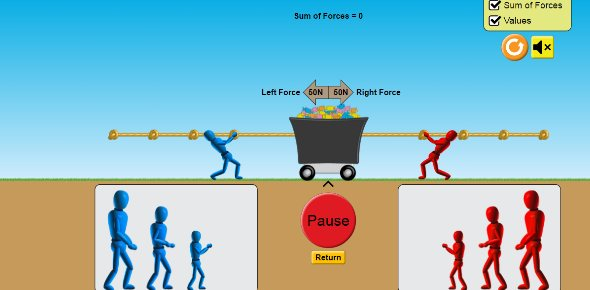# Force And Motion

5 Questions | Total Attempts: 1114SettingsRelated Topics
• 1.
When 2 forces are not equil what kind of forces is it ?
• A.

Unbalanced

• B.

Balance

• C.

Push

• D.

Pull

• 2.
Which is a change in velocity?
• A.

Speed

• B.

Velocity

• C.

Acceleration

• 3.
A balanced force is
• A.

When two objects act on each other and they both go one way .

• B.

When two objects act on each other and stay in the same spot .

• 4.
What is speed in a given direction?
• A.

Speed

• B.

Acceleration

• C.

Velocity

• 5.
How fast something is moving is its ?
• A.

Speed

• B.

Velocity

• C.

Acceleration